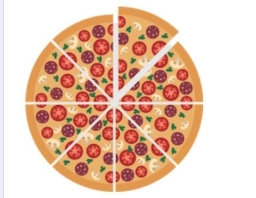# Mary needs

Mary needs to order pizza for 18 students. Each student should get ¼ of a pizza. How many pizzas should Mary order?

n =  4 12 = 9/2

### Step-by-step explanation:Did you find an error or inaccuracy? Feel free to write us. Thank you!

Tips for related online calculators
Need help calculating sum, simplifying, or multiplying fractions? Try our fraction calculator.
Need help with mixed numbers? Try our mixed-number calculator.